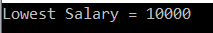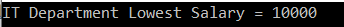# Linq Min Method in C#

## Linq Min Method in C# with Examples

In this article, I am going to discuss the Linq Min Method in C# with examples. Please read our previous article before proceeding to this article where we discussed the Linq Max Aggregate Functions in C# with some examples. As part of this article, we are going to discuss the following pointers.

1. What is Linq Min Method in C#?
2. Multiple examples using both Method and Query syntax.
##### What is Linq Min Method in C#?

The Linq Min method is used to returns the lowest numeric value from the collection on which it is applied. Let us understand the Min() method with some examples.

##### Example1:

The following example returns the lowest number from the collection.

```using System;
using System.Linq;
namespace LINQDemo
{
class Program
{
static void Main(string[] args)
{
int[] intNumbers = new int[] { 60, 80, 50, 90, 10, 30, 70, 40, 20, 100 };

//Using Method Syntax
int MSLowestNumber = intNumbers.Min();

//Using Query Syntax
int QSLowestNumber = (from num in intNumbers
select num).Min();

Console.WriteLine("Lowest Number = " + MSLowestNumber);

}
}
}
```

Once you run the application, it will display the output as 10.

Note: We don’t have any operator called Min in Linq to write the query syntax. So here we need to use the mixed syntax.

##### Example2: Min with filter

Now we need to return the lowest number from the collection where the number is greater than 50.

```using System;
using System.Linq;
namespace LINQDemo
{
class Program
{
static void Main(string[] args)
{
int[] intNumbers = new int[] { 60, 80, 50, 90, 10, 30, 70, 40, 20, 100 };

//Using Method Syntax
int MSLowestNumber = intNumbers.Where(num => num > 50).Min();

//Using Query Syntax
int QSLowestNumber = (from num in intNumbers
where num > 50
select num).Min();

Console.WriteLine("Lowest Number = " + MSLowestNumber);
}
}
}
```

It will print the output as 60.

##### Example3: Min Method with Predicate

Instead of using the where method to filter the data, you can also use the other overloaded version of the Min method which takes one Predicate and within that predicate, you can write your logic to filter the data as shown in the below example.

```using System;
using System.Linq;
namespace LINQDemo
{
class Program
{
static void Main(string[] args)
{
int[] intNumbers = new int[] { 60, 80, 50, 90, 10, 30, 70, 40, 20, 100 };

//Using Method Syntax
int MSLowestNumber = intNumbers.Where(num => num > 50).Min(num => {
if (num > 50)
return num;
else
return 0;
});

Console.WriteLine("Lowest Number = " + MSLowestNumber);
}
}
}
```

It should give the same output as the previous example.

##### Working with Complex Type:

We are going to work with the following Employee class.

```using System.Collections.Generic;
namespace LINQDemo
{
public class Employee
{
public int ID { get; set; }
public string Name { get; set; }
public int Salary { get; set; }
public string Department { get; set; }

public static List<Employee> GetAllEmployees()
{
List<Employee> listStudents = new List<Employee>()
{
new Employee{ID= 101,Name = "Preety", Salary = 10000, Department = "IT"},
new Employee{ID= 102,Name = "Priyanka", Salary = 15000, Department = "Sales"},
new Employee{ID= 103,Name = "James", Salary = 50000, Department = "Sales"},
new Employee{ID= 104,Name = "Hina", Salary = 20000, Department = "IT"},
new Employee{ID= 105,Name = "Anurag", Salary = 30000, Department = "IT"},
new Employee{ID= 106,Name = "Sara", Salary = 25000, Department = "IT"},
new Employee{ID= 107,Name = "Pranaya", Salary = 35000, Department = "IT"},
new Employee{ID= 108,Name = "Manoj", Salary = 11000, Department = "Sales"},
new Employee{ID= 109,Name = "Sam", Salary = 45000, Department = "Sales"},
new Employee{ID= 110,Name = "Saurav", Salary = 25000, Department = "Sales"}
};

return listStudents;
}
}
}
```

In the above Employee class, we define four properties such as ID, Name, Salary, and Department along with the GetAllEmployees() which will return the list of all the employees.

##### Example4:

The following example returns the lowest salary among all the employees.

```using System;
using System.Linq;
namespace LINQDemo
{
class Program
{
static void Main(string[] args)
{
//Using Method Syntax
var MSLowestSalary = Employee.GetAllEmployees()
.Min(emp => emp.Salary);

//Using Query Syntax
var QSLowestSalary = (from emp in Employee.GetAllEmployees()
select emp).Min(e => e.Salary);

Console.WriteLine("Lowest Salary = " + MSLowestSalary);
}
}
}
```

Output:##### Example5:

The following example returns the Lowest Salary of IT department.

```using System;
using System.Linq;
namespace LINQDemo
{
class Program
{
static void Main(string[] args)
{
//Using Method Syntax
var MSLowestSalary = Employee.GetAllEmployees()
.Where(emp => emp.Department == "IT")
.Min(emp => emp.Salary);

//Using Query Syntax
var QSLowestSalary = (from emp in Employee.GetAllEmployees()
where emp.Department == "IT"
select emp).Min(e => e.Salary);

Console.WriteLine("IT Department Lowest Salary = " + QSLowestSalary);